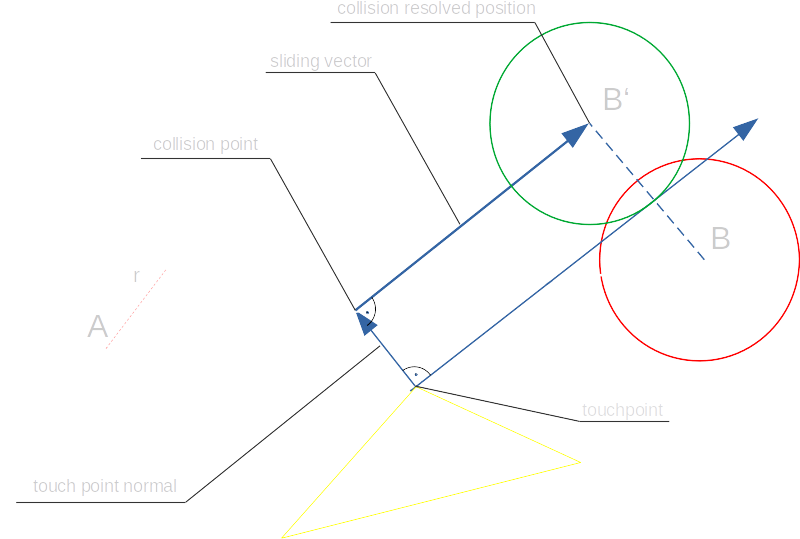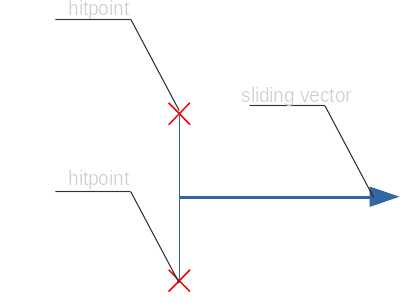Continuous Collision Detection:

# Swept Sphere - Triangle

## Scenario

For a 3D gaming project I needed to test for collisions between a moving character and its environment to prevent the character from falling through the floor or walking through obstacles in the World.

In general there are two ways to approach this problem: discrete collision detection and continuous collision detection. The first checks only at specific positions of a character whether it is in a collision or not, the second checks for a collision when it tries to move from a position A to a position B and handles all collisions on the way.

I implemented the second approach since it is independent of the frame rate and therefore a more robust solution.

#### Overview

This image shows an overview over the process of a single collision detection step. It may look a bit convoluted, but can be understood easily when observed step by step: Starting at point "A" the sphere touches the triangle at the "collision point" (dashed sphere). The sphere slides along the "sliding vector" which is orthogonal to the "touch point normal". The length of the sliding vector is determined by projecting the original target point "B" onto the sliding vector.## The Basic Algorithm

1. Make sure we have no collision with anything at point A. If there is, move to the last known position that was not in collision.
2. Search for the earliest collision with any triangle and determine the t value of collision, the touch point and the normal vector at the touch point.
Remove the direction of the impact normal from the velocity vector - we've hit an obstacle and need to stop moving into the direction this obstacles prevented us from going. This automatically enables us to determine the force of impact - in case it is needed.
3. Calculate the new target position using the sliding vector.
4. Repeat this process until no more collisions are found or 2 iterations have been done.

If the above steps have been run 2 iterations, we have 2 normals of points we ran into in a single movement. This means the player needs to slide against two hitpoints. If a sphere hits two points, it can only slide perpendicular to the connecting line of the two points. So in this case we calculate a slide along the vector orthogonal to the line connecting the two hitpoints.
The algorithm above is then executed again for the new movement.We end the collision detection and return the last safe position found when...

• The movement has (nearly) stopped - means: The collision resolved points are extremely close to the last position. This is not worth the effort.
• The velocity we adjust on impact points back to the direction we came from.
• We collide with more than 2 points, which means there is no more degree of freedom to move to

## Pseudo-Distance Function

To determine whether a sphere is in collision with a triangle, I'm using a pseudo-distance function that calculates the shortest distance between a sphere and a triangle. This function returns

• < 0 if the sphere and triangle intersect
• 0 if the sphere and triangle touch each other
• > 0 if the sphere and triangle are separated
The big advantage of having such a function is that we can use standard numerical algorithms to find the root and therefore the point of intersection. And by using just a different pseudo-distance function arbitrary objects can be checked for collision against each other.

## Common Pitfalls

Using a different function to determine whether a collision is happening at the starting position than the one used to resolve a collision.

In this case the precision for the collision check function is different from the one that thinks can safely move the sphere to a collision-free space. This yields to inconsistencies between both calculations and in the worst case to a lot of collision-at-start errors popping up that need to be handled appropriately.

Getting too close to a collision results in problems due to floating point precision limits.

In general the collision detection code should always contain some epsilon value to allow for a bit of relaxed calculation. It is enough to consider a distance to a triangle of 1e-5 units to be close enough for being in a collision.

Assuming that sliding is precise

When the sphere slides along the calculated vector it can happen, that because of precision limits this vector points a tiny bit towards the plane, making the collision even closer than the distance at which the point of contact was calculated. This may result in the sphere getting closer and closer to the surface until we run into precision problems again. In my code I add a tiny bit of normal direction to the sliding vector to make sure it does not move closer to the plane.